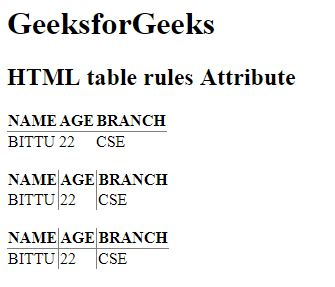Related Articles

# HTML | <table> rules Attribute

• Last Updated : 29 May, 2019

The HTML <table> rules Attribute is used to specify the which parts of the inside borders that should be visible.

Syntax:

`<table rules="value">`

Attribute Values:

• none: It does not create any lines.
• groups: It create lines between row and column groups.
• rows: It creates line between the rows.
• cols: It creates line between the columns.
• all: It creates line between the rows and columns.

Note: The <table> rules Attribute is not supported by HTML 5.

Example:

 ```<``html``>`` ` `<``head``>``    ``<``title``>``        ``HTML table rules Attribute``    `````` ` `<``body``>``    ``<``h1``>GeeksforGeeks`` ` `    ``<``h2``>HTML table rules Attribute`` ` `    ``<``table` `rules``=``"rows"``>``        ``<``tr``>``            ``<``th``>NAME``            ``<``th``>AGE``            ``<``th``>BRANCH``        ````        ``<``tr``>``            ``<``td``>BITTU``            ``<``td``>22``            ``<``td``>CSE``        ````    ````    ``<``br``>``    ``<``table` `rules``=``"cols"``>``        ``<``tr``>``            ``<``th``>NAME``            ``<``th``>AGE``            ``<``th``>BRANCH``        ````        ``<``tr``>``            ``<``td``>BITTU``            ``<``td``>22``            ``<``td``>CSE``        ````    ````    ``<``br``>``    ``<``table` `rules``=``"all"``>``        ``<``tr``>``            ``<``th``>NAME``            ``<``th``>AGE``            ``<``th``>BRANCH``        ````        ``<``tr``>``            ``<``td``>BITTU``            ``<``td``>22``            ``<``td``>CSE``        ````    `````` ` ``

Output:Supported Browsers: The browser supported by HTML <table> rules Attribute are listed below: